In: Chemistry

# Assign formal charges to each atom in the O3 molecule shown here

Assign formal charges to each atom in the O3 molecule shown here. Be sure to click the +  or - button before clicking on the molecule.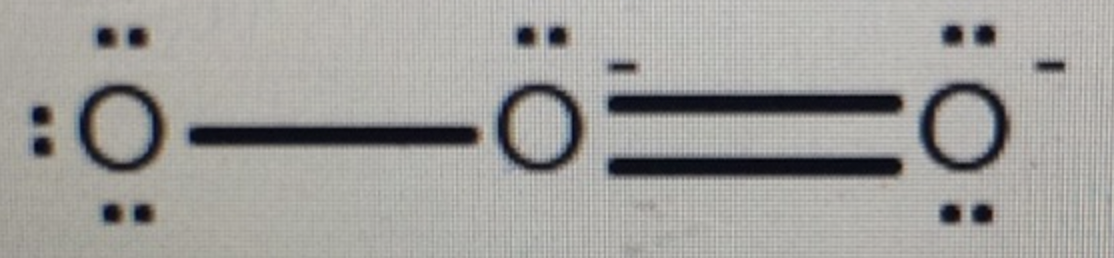## Solutions

##### Expert Solution

Concepts and reason

The concept used to solve this problem is the formal charge calculation of the oxygen atoms present in the ozone molecule.

Fundamentals

A charge that is assigned to an atom, which is present in the molecule, is represented as a formal charge. In two bonded atoms, a formal charge assumes that the electrons are equally shared between them. The formal charge is calculated using the following formula:

Formal charge $$=\mathrm{V}-\mathrm{N}-\frac{\mathrm{B}}{2}$$

Here, $$\mathrm{V}$$ is the number of valence electrons, $$\mathrm{N}$$ is the number of non-bonding electrons and $$\mathrm{B}$$ is the number of bonding electrons.

The structure is as follows:

Calculate the formal charge for the circled oxygen in ozone molecule as follows: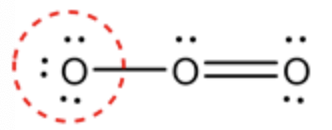Formal charge $$=\mathrm{V}-\mathrm{N}-\frac{\mathrm{B}}{2}$$

\begin{aligned}=& 6-6-\frac{2}{2} \\ &=-1 \end{aligned}

Explanation | Common mistakes | Hint for next step

The marked oxygen atom has 6 valence electrons. It contains 6 non-bonding electrons. The marked oxygen atom is bonded with one oxygen atom. Therefore, the bonding electron of oxygen is $$2 .$$ Substitute the values in a formal charge formula to determine the formal charge of oxygen (marked).

The structure is as follows: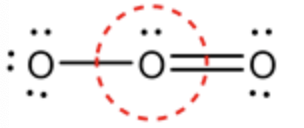Calculate the formal charge for the circled oxygen in ozone molecule as follows:

Formal charge $$=\mathrm{V}-\mathrm{N}-\frac{\mathrm{B}}{2}$$

$$=6-2-\frac{6}{2}$$

$$=+1$$

Explanation | Common mistakes | Hint for next step

The oxygen atom in the middle has 6 valence electrons and has 2 non-bonding electrons. The central oxygen atom is bonded with 1 double bond and 1 single bond. So, there is a total of 3 bonds containing 6 electrons (bonding electrons). The formal charge of the middle oxygen atom can be determined by substituting the values in a formal charge formula.

The structure is as follows: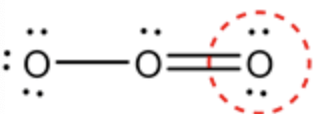Calculate the formal charge for the circled oxygen in ozone molecule as follows:

Formal charge $$=\mathrm{V}-\mathrm{N}-\frac{\mathrm{B}}{2}$$

$$=6-4-\frac{4}{2}$$

$$=0$$

The formal charge of each oxygen atom in $$\mathrm{O}_{3}$$ molecule are labeled as follows: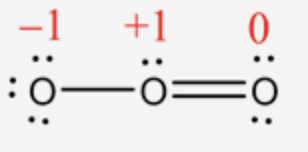The oxygen atom present on the right side contains 6 valence electrons which have 2 non-bonded electrons. The marked oxygen is bonded with 1 double bond with a central oxygen atom. So, the number of bonding electrons is $$4 .$$ The formal charge of the corner oxygen atom (right corner) can be determined by substituting the values in the formal charge formula.

The formal charge of each oxygen atom in $$\mathrm{O}_{3}$$ molecule are labeled as follows: